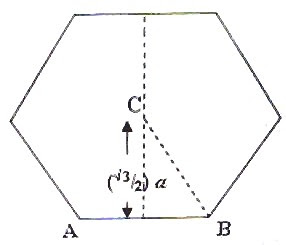## Monday, September 22, 2008

### Moment of inertia - Two Multiple Choice Questions

The value of a man resides in what he gives and not in what he is capable of receiving

– Albert Einstein

You will find many questions involving moment of inertia on this site and you can access all of them by clicking on the label ‘MOMENT OF INERTIA’ below this post. I give you two more questions here, the first being the one which appeared in the AIEEE 2008 question paper. You will have to apply the parallel axis theorem to answer these questions.

(1) Consider a uniform square plate of side ‘a’ and mass ‘m’. The moment of inertia of this plate about an axis perpendicular to its plane and passing through one of its corners is

(1) (5/6) ma2

(2) (1/12) ma2(3) (7/12) ma2

(4) (2/3) ma2

The moment of inertia (ICM) of the square plate about an axis through its centre of mass (CM) passing perpendicular to its plane is given by

ICM = m(a2 + a2)/12 = ma2/6.

[Note that generally for a bar of rectangular cross section, the moment of inertia about an axis passing through the centre, perpendicular to its length is m(l2 + b2)12 where l is the length and b is the breadth].

The moment of inertia (I) about a parallel axis through one corner O is given by

I = ICM + m(a/√2)2 since the corner is at a distance a/√2 from the centre of mass (fig).

Thus I = ma2/6 + ma2/2 = (2/3) ma2

(2) A thin uniform rod of mass 6m is bent to form a regular hexagon of side a. What is the moment of inertia of the hexagon about an axis passing through its centre and perpendicular to its plane?(a) 5 ma2

(b) 5 ma2/6

(c) 5 ma2/3

(d) 6 ma2

(e) 6 ma2/5

Consider one side (such as AB) of the hexagon. Since its mass is m and its length is a, its moment of inertia about an axis through its centre and perpendicular to its length is given by

ICM = ma2/12.

The moment of inertia of this side about a parallel axis through the centre C of the hexagon is given by

I = ICM + m[(√3/2)a] 2 since the side is at a distance (√3/2)a from the centre of the hexagon (fig).

Thus I = ma2/12 + 3ma2/4 = 5 ma2/6.

Since there are six sides, the total moment of inertia of the hexagon is 6×5 ma2/6 = 5 ma2.